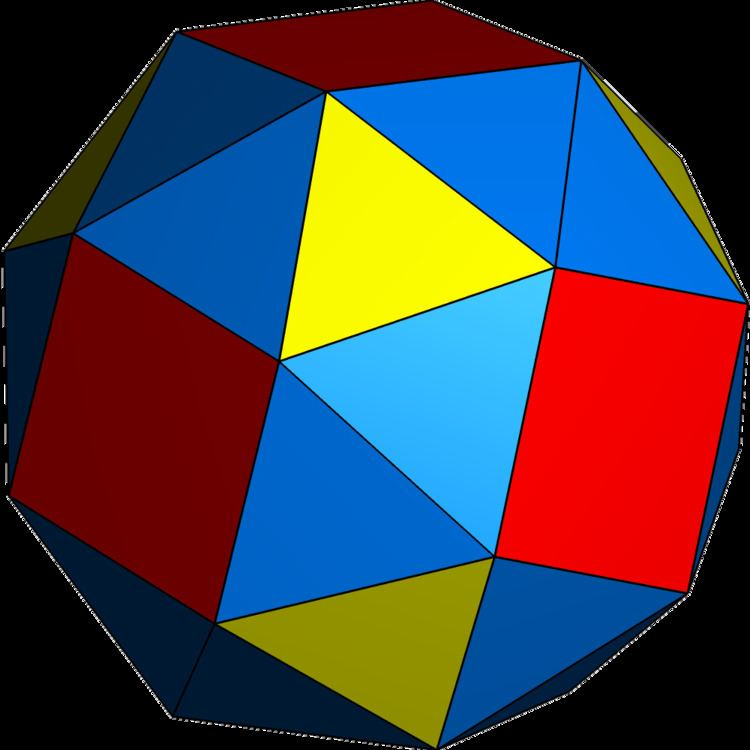# Snub (geometry)

Updated on
Covid-19In geometry, a snub is an operation applied to a polyhedron. The term originates from Kepler's names of two Archimedean solids, for the snub cube (cubus simus) and snub dodecahedron (dodecaedron simum). In general, snubs have chiral symmetry with two forms, with clockwise or counterclockwise orientations. By Kepler's names, a snub can be seen as an expansion of a regular polyhedron, with the faces moved apart, and twists on their centers, adding new polygons centered on the original vertices, and pairs of triangles fitting between the original edges.

## Contents

The terminology was generalized by Coxeter, with a slightly different definition, for a wider set of uniform polytopes.

## Conway snubs

John Conway explored generalized polyhedron operators, defining what is now called Conway polyhedron notation, which can be applied to polyhedra and tilings. Conway calls Coxeter's operation a semi-snub.

In this notation, snub is defined by the dual and gyro operators, as s = dg, and it is equivalent to an alternation of a truncation of an ambo operator. Conway's notation itself avoids Coxeter's alternation (half) operation since it only applies for polyhedra with only even-sided faces.

In 4-dimensions, Conway suggests the snub 24-cell should be called a semi-snub 24-cell because it doesn't represent an alternated omnitruncated 24-cell like his 3-dimensional polyhedron usage. It is instead actually an alternated truncated 24-cell.

## Coxeter's snubs, regular and quasiregular

Coxeter's snub terminology is slightly different, meaning an alternated truncation, deriving the snub cube as a snub cuboctahedron, and the snub dodecahedron as a snub icosidodecahedron. This definition is used in the naming two Johnson solids: snub disphenoid, and snub square antiprism, as well as higher dimensional polytopes such as the 4-dimensional snub 24-cell, or s{3,4,3}.

A regular polyhedron (or tiling) with Schläfli symbol, { p , q } , and Coxeter diagram , has truncation defined as t { p , q } , and and snub defined as an alternated truncation h t { p , q } = s { p , q } , and Coxeter diagram . This construction requires q to be even.

A quasiregular polyhedron { p q } or r{p,q}, with Coxeter diagram or has a quasiregular truncation defined as t { p q } or tr{p,q}, and Coxeter diagram or and quasiregular snub defined as an alternated truncated rectification h t { p q } = s { p q } or htr{p,q} = sr{p,q}, and Coxeter diagram or .

For example, Kepler's snub cube is derived from the quasiregular cuboctahedron, with a vertical Schläfli symbol { 4 3 } , and Coxeter diagram , and so is more explicitly called a snub cuboctahedron, expressed by a vertical Schläfli symbol s { 4 3 } and Coxeter diagram . The snub cuboctahedron is the alternation of the truncated cuboctahedron, t { 4 3 } and .

Regular polyhedra with even-order vertices to also be snubbed as alternated trunction, like a snub octahedron, s { 3 , 4 } , (and snub tetratetrahedron, as s { 3 3 } , ) represents the pseudoicosahedron, a regular icosahedron with pyritohedral symmetry. The snub octahedron is the alternation of the truncated octahedron, t { 3 , 4 } and , or tetrahedral symmetry form: t { 3 3 } and .

Coxeter's snub operation also allows n-antiprisms to be defined as s { 2 n } or s { 2 , 2 n } , based on n-prisms t { 2 n } or t { 2 , 2 n } , while { 2 , n } is a regular n-hosohedron, a degenerate polyhedron, but a valid tiling on the sphere with digon or lune-shaped faces.

The same process applies for snub tilings:

## Nonuniform snub polyhedra

Nonuniform polyhedra with all even-valance vertices can be snubbed, including some infinite sets, for example:

## Coxeter's uniform snub star-polyhedra

Snub star-polyhedra are constructed by their Schwarz triangle (p q r), with rational ordered mirror-angles, and all mirrors active and alternated.

## Coxeter's higher-dimensional snubbed polytopes and honeycombs

In general, a regular polychora with Schläfli symbol, { p , q , r } , and Coxeter diagram , has a snub with extended Schläfli symbol s { p , q , r } , and .

A rectified polychora { p q , r } = r{p,q,r}, and has snub symbol s { p q , r } = sr{p,q,r}, and .

## Examples

There is only one uniform snub in 4-dimensions, the snub 24-cell. The regular 24-cell has Schläfli symbol, { 3 , 4 , 3 } , and Coxeter diagram , and the snub 24-cell is represented by s { 3 , 4 , 3 } , Coxeter diagram . It also has an index 6 lower symmetry constructions as s { 3 3 3 } or s{31,1,1} and , and an index 3 subsymmetry as s { 3 3 , 4 } or sr{3,3,4}, and or .

The related snub 24-cell honeycomb can be seen as a s { 3 , 4 , 3 , 3 } or s{3,4,3,3}, and , and lower symmetry s { 3 3 , 4 , 3 } or sr{3,3,4,3} and or , and lowest symmetry form as s { 3 3 3 3 } or s{31,1,1,1} and .

A Euclidean honeycomb is an alternated hexagonal slab honeycomb, s{2,6,3}, and or sr{2,3,6}, and or sr{2,3}, and .

Another Euclidean (scaliform) honeycomb is an alternated square slab honeycomb, s{2,4,4}, and or sr{2,41,1} and :

The only uniform snub hyperbolic uniform honeycomb is the snub hexagonal tiling honeycomb, as s{3,6,3} and , which can also be constructed as an alternated hexagonal tiling honeycomb, h{6,3,3}, . It is also constructed as s{3[3,3]} and .

Another hyperbolic (scaliform) honeycomb is an snub order-4 octahedral honeycomb, s{3,4,4}, and .

## References

Similar Topics
Art School Confidential (film)
Alonzo (rapper)
Nat Muir
Topics